﻿ Synergic Effect of Post-Fired Volume Shrinkage and Porosity of Bricks on Its Water Absorption Level during Service in Submerged State

### Synergic Effect of Post-Fired Volume Shrinkage and Porosity of Bricks on Its Water Absorption Level ...

C. I. Nwoye, I. E. Nwosu, S. O. NwakpaOPEN ACCESSPEER-REVIEWED

## Synergic Effect of Post-Fired Volume Shrinkage and Porosity of Bricks on Its Water Absorption Level during Service in Submerged State

C. I. Nwoye1,, I. E. Nwosu2, S. O. Nwakpa1

1Department of Metallurgical and Materials Engineering, Nnamdi Azikiwe University, Awka, Nigeria

2Department of Environmental Engineering, Federal University of Technology, Owerri, Nigeria

### Abstract

The synergic effect of post-fired volume shrinkage and apparent porosity of bricks on its water absorption level during service in submerged state was evaluated. A clay sample was prepared and processed, following a well detailed step-wise route. Analysis of water absorption by the produced bricks while serving under submerged condition was carried out using a two-factorial empirical model expressed as ξ = - 1.0525 ₰ – 2.6392 ϑ + 107.6801. The validity of the derived model was rooted in the core expression ξ – 107.6801 = - 1.0525 ₰ – 2.6392 ϑ where both side of the expression correspondingly approximately equal. Results generated from both experiment and model prediction indicates that water absorption decreases with decreasing apparent porosity and increasing post-fired volume shrinkage. Evaluated results indicated that the correlations between water absorption and post-fired volume shrinkage & apparent porosity and the standard error incurred in predicting water absorption for each value of the post-fired volume shrinkage & apparent porosity considered, as obtained from experiment, derived model and regression model were all > 0.95 as well as 0.0704, 0.0693 and 4.38 x 10-5 & 0.0028, 0.2201 and 2.58 x 10-5 % respectively. The maximum deviation of the model-predicted water absorption (from experimental results) was less than 8%. This translated into over 92% operational confidence for the derived model as well as over 0.92 synergic effective coefficients for the dependence of water absorption level of the submerged bricks on post-fired volume shrinkage & apparent porosity.

### At a glance: Figures

1234
Prev Next

• Nwoye, C. I., I. E. Nwosu, and S. O. Nwakpa. "Synergic Effect of Post-Fired Volume Shrinkage and Porosity of Bricks on Its Water Absorption Level during Service in Submerged State." International Journal of Materials Lifetime 1.1 (2014): 13-19.
• Nwoye, C. I. , Nwosu, I. E. , & Nwakpa, S. O. (2014). Synergic Effect of Post-Fired Volume Shrinkage and Porosity of Bricks on Its Water Absorption Level during Service in Submerged State. International Journal of Materials Lifetime, 1(1), 13-19.
• Nwoye, C. I., I. E. Nwosu, and S. O. Nwakpa. "Synergic Effect of Post-Fired Volume Shrinkage and Porosity of Bricks on Its Water Absorption Level during Service in Submerged State." International Journal of Materials Lifetime 1, no. 1 (2014): 13-19.

 Import into BibTeX Import into EndNote Import into RefMan Import into RefWorks

### 1. Introduction

The incessant collapse of buildings in the riverine areas especially in Nigeria, and also in other environments due to stagnation of water around buildings (as a result of flood) has raised an unavoidable need to produce building materials such as bricks which would have low water absorption capacity (low porosity) to avoid abrupt failure.

Research  has shown that pores affect the strength of ceramics adversely; on one hand by reducing the cross-sectional area over which load is applied, and on the other hand acting as stress concentrators.

Durability of buildings constructed with bricks requires these bricks to have good structural stability through improvements in its chemical, physical and mechanical properties.

Studies [2-7] have shown that ceramics can be moulded into different shapes for different uses depending on the forming techniques employed.

Several works [1, 8, 9, 10] have been carried out on shrinkage of clay during drying. In all these works, porosity was shown to influence the swelling and shrinkage behaviour of clay products of different geometry. Past research  shows that drying occurs in three stages; increasing rate, constant and decreasing rate. The researcher pointed out that during the increasing rate; evaporation rate is higher than evaporating surface hence more water is lost. At constant rate, the evaporation rate and evaporation surface are constant. Report of the research posited that shrinkage occurs at this stage.

Similar studies  also suggested that at this stage, free water is removed between the particles and the inter-particle separation decreases, resulting in shrinkage. During the decreasing rate, particles make contacts as water is removed, which causes shrinkage to cease.

Report  has shown that fine particles shrink more, are denser and exhibit excellent mechanical properties. The report also indicated an evaluation of the relationship between particle size and size distribution with linear drying shrinkage. Results generated from the evaluation revealed that firing shrinkage and apparent porosity have no visible relationship with particle size and linear drying shrinkage. It was therefore concluded based on these results that the finer the particle size, the lesser the apparent porosity and greater the bulk density.

The volume shrinkage resulting from the initial air-drying of wet clay has been successfully calculated using a derived model . The model;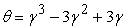(1)

calculates the volume shrinkage θ when the value of dried shrinkage γ, experienced during air-drying of wet clays is known. The model was found to be third-order polynomial in nature. Olokoro clay was found to have the highest shrinkage during the air drying condition, followed by Ukpor clay while Otamiri clay has the lowest shrinkage. Volume shrinkage was discovered to increase with increase in dried shrinkage until maximum volume shrinkage was reached, hence a direct relationship.

Overall volume shrinkage in molded clay products (from initial air-drying stage to completion of firing at a temperature of 1200°C), have also been predicted  using a model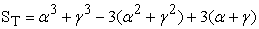(2)

Comparative analysis of results of the overall volume shrinkage predicted by the model and those evaluated from the conventional equations indicated proximate agreement. The overall volume shrinkage was found to depends on direct values of the dried γ and fired shrinkage α for its precision. Overall volume shrinkage was found to increase with increase in dried and fired shrinkages until overall volume shrinkage reaches maximum.

Successful predictability of the Post-Fired Volume Shrinkage (PFVS) has been carried out  based on its apparent porosity (AP) and water absorption capacity (WAC). Prediction of the PFVS was carried out using a two-factorial empirical model expressed as;(3)

Where ϑ = PFVS, ₰ = AP and ξ = WAC. The correlations between PFVS and WAC & apparent porosity as obtained from experiment, derived model and regression model were all > 0.97. The maximum deviation of the model-predicted water absorption (from experimental results) was less than 5.57%. This translated into over 94% operational confidence for the derived model as well as over 0.94 effective response coefficients of WAC and apparent porosity to PFVS of the bricks.

The present work aims at evaluating the synergic effect of post-fired volume shrinkage and apparent porosity of bricks on its water absorption level during service in submerged state

### 2. Materials and Method

The materials used for this research work includes Olokoro Clay mined at Umuahia, Imo and Bentonite obtained from Bridge Head Market, Onitsha, Anambra state, Nigeria. The chemical composition of the clay used is shown in Table 1.

Figure 1. (a) Bentonite powder (b) Olokoro clay (as mined) (c) Dried Olokoro clay (d) Dried Olokoro clay mixed with Bentonite
2.1. Clay Sizing and Moulding

Size analysis of the clay was carried out using an assembly of sieves having opening as 100, 300 and 1000 μm in ascending order. The sieve assembly was placed on a mechanical sieve shaker and power supply switched on. The set-up was allowed to function for 4 minutes. Particle sizes lesser than 100 μm were taken as fine particle size (A), those at 100 μm were taken as less finer (B), those lesser than 300 μm were taken as medium particle size (C), those at 300 μm were taken as enhanced medium particle size (D) while particle size within range 300-1000 μm were taken as coarse particle size (E). The sieving process was repeated severally until the quantity of clay required for the moulding process was available. Hundred grams (100g) of the sieved clay sample and 10g of bentonite powder were weighed out and thoroughly mixed. To increase the plasticity and strength of the clay material during firing, bentonite powder was added. Six percent (6%) of total weight (clay and Bentonite) of water was added and it was then mixed until complete homogeneity was achieved. The mixed samples were poured into the rectangular metal mould of internal dimension 50 x 18 x 10 mm. The green samples were marked immediately after moulding with two parallel lines (along the length) 70 mm apart. The distance between these lines is L

2.2. Air Drying

The moulded specimens were carefully placed in a plastic tray and kept outside the laboratory to loose some water and become strengthened. The reason for air drying includes (1) to prevent the samples from being defective as a result of evaporation during oven drying and firing (2) to give the specimens adequate strength during oven drying and firing.

2.3. Oven Drying and Firing

An electrically heated oven of internal dimension 500 x 500mm was used in the drying operation. The oven was sourced from Erosion Research Center of the Federal University of Technology, Owerri (FUTO). Each set of the specimens was dried at a temperature of 125°C for 1hr, after which their respective weights were measured. The clay samples were then charged into an electric kiln and heated at a lower temperature 125°C, after which the temperature was increased and fired at 1200°C for 48 hrs. The samples were cooled in the furnace for 48hrs after firing. The distance between the two parallel lines was determined after oven drying L1 and firing L2.

2.4. Determination of Post-Fired Volume Shrinkage, Apparent Porosity and Water Absorption

Post-fired volume shrinkage V, was calculated using the formular: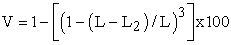(4)

Where

L = Original length (mm)

L1= Dry length (mm)

L2= Fired length (mm)

Apparent porosity and water absorption were determined using the conventional standard technique .

### 3. Results and Discussion

The result of chemical analysis of Olokoro clay is shown in Table 1. The table shows that the clay is most constituted by SiO2 and then Al2O3 while Na2O is the poorest constituent.

#### Table 2. Variation of water absorption with post-fired volume shrinkage and apparent porosity

Table 2 shows that water absorption decreases with decreasing apparent porosity and increasing post-fired volume shrinkage. This was so because during firing, water present in the clay was significantly removed, simultaneously accompanied by enhanced decrease in the inter-particle spacing, leading to increased post-fired volume shrinkage in accordance with past findings .

It is strongly believed that a good knowledge of the empirical relationship between the quantity of water absorbed (by the brick in submerged condition) and the post-fired volume shrinkage & apparent porosity gives a structural engineer an idea of what to expect in terms of water absorption of the bricks (when used for building in easily flood environment) for any choice of clay particle size inculcated in producing bricks for building. Table 2 shows that increase in the clay grain size results to increase in the water absorption of the produced bricks due to increase in the apparent porosity and decrease in the post-fired volume shrinkage. This is also in line with past research .

3.1. Model Formulation

Experimental data generated from this research work were used for the model formulation. Computational analysis of the data shown in Table 2, gave rise to Table 3 which indicate that;(5)

Introducing the values of K, S, and N into equation (5) reduces it to;(6)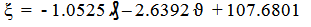(7)

Where

(ϑ) = Post fired volume shrinkage (%)

(₰) = Apparent porosity (%)

(ζ ) = Water absorption (%)

S = 107.6801, K = 1.0525, and N = 2.6392 These are empirical constants (determined using C-NIKBRAN .

### 4. Boundary and Initial Condition

Consider a rectangular shaped clay product of length 49mm, width 17mm, and breadth 9mm exposed to drying in the oven while it was in slight wet condition and then fired in the furnace. Initially, atmospheric levels of oxygen are assumed. Atmospheric pressure was assumed to be acting on the clay samples during the drying process (since the furnace is not air-tight). The sizes of clay particles used were < 100, 100-300 and 300-1000μm while weights of clay and binder (bentonite) used (for each rectangular product) were 100g and 10g respectively. Quantity of water used for mixing was 6% (of total weight). Oven drying and firing temperatures used were 125 and 1200°C for 1and 48 hrs respectively. Area of evaporating surface was 833mm2. Cooling time for samples was 48 hrs.

The boundary conditions are: atmospheric levels of oxygen at the top and bottom of the clay samples since they are dried under the atmospheric condition. No external force due to compression or tension was applied to the drying clays. The sides of the particles and the rectangular shaped clay products are taken to be symmetries.

4.1. Model Validation

Equation (7) is the derived model. The validity of the model is strongly rooted on equation (6) where both sides of the equation are correspondingly approximately equal. Table 3 also agrees with equation (6) following the values of ξ - 107.6801 and - 1.0525₰ - 2.6392 ϑ evaluated from the experimental results in Table 2.

#### Table 3. Variation of ξ - 107.6801 with - 1.0525₰ - 2.6392 ϑ

Furthermore, the derived model was validated by comparing the model-predicted water absorption and that obtained from the experiment. This was done using the 4th Degree Model Validity Test Techniques (4th DMVTT); statistical graphical, computational and deviational analysis.

4.2. Statistical Analysis
4.2.1. Standard Error (STEYX)

The standard errors incurred in predicting water absorption for each value of the volume shrinkage & apparent porosity considered as obtained from experiment and derived model were 0.0704 and 0.0693 & 0.0028 and 0.2201 % respectively. The standard error was evaluated using Microsoft Excel version 2003.

4.2.2. Correlation (CORREL)

The correlation coefficient between water absorption and volume shrinkage & apparent porosity were evaluated from the results of the derived model and experiment, considering the coefficient of determination R2 from Figure 2 – Figure 5. The evaluation was done using Microsoft Excel version 2003.(8)

The evaluated correlations are shown in Table 4 and Table 5. These evaluated results indicate that the derived model predictions are significantly reliable and hence valid considering its proximate agreement with results from actual experiment.

#### Table 5. Comparison of the correlation evaluated from derived model-predicted ExD based on apparent porosity

4.3. Graphical Analysis

Comparative graphical analysis of Figure 6 and Figure 7 show very close alignment of the curves from the experimental (ExD) and model-predicted (MoD) water absorptions.

Furthermore, the degree of alignment of these curves is indicative of the proximate agreement between both experimental and model-predicted water absorption.

4.4. Comparison of derived model with standard model

The validity of the derived model was also verified through application of the regression model (Reg) (Least Square Method using Excel version 2003) in predicting the trend of the experimental results.

Figure 8. 3-D Effects comparison of water absorptions (relative to volume shrinkage) as obtained from experiment, derived model and regression model

Comparative analysis of Figure 8 and Figure 9 shows very close alignment of curves and areas covered by water absorptions, which precisely translated into significantly similar trend of data point’s distribution for experimental (ExD), derived model (MoD) and regression model-predicted (ReG) results of water absorption.

Also, the calculated correlations (from Figure 8 and Figure 9) between water absorption and volume shrinkage & apparent porosity for results obtained from regression model gave 1.0000 & 1.0000 respectively. These values are in proximate agreement with both experimental and derived model-predicted results. The standard errors incurred in predicting water absorption for each value of the volume shrinkage & apparent porosity considered as obtained from regression model were 4.38 x 10-5 and 2.58 x 10-5 % respectively.

Figure 9. Comparison of water absorptions (relative to apparent porosity) as obtained from experiment, derived model and regression model
4.5. Deviational Analysis

The deviation Dv, of model-predicted water absorption from the corresponding experimental result was given by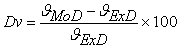(9)

Where ζExD and ζMoD are water absorptions obtained from experiment and derived model respectively.

Critical analysis of the water absorption obtained from experiment and derived model shows low deviations on the part of the model-predicted values relative to values obtained from the experiment. This was attributed to the fact that the surface properties of clay and the physico-chemical interactions between the clay and the binder which played vital roles during water absorption were not considered during the model formulation. This necessitated the introduction of correction factor, to bring the model-predicted water absorption level to those of the corresponding experimental values.

Figure 11. Variation of deviation with water absorption (relative to apparent porosity)

Deviational analysis from Figure 10 and Figure 11 indicates that the precise maximum deviation of model-predicted water absorption from the experimental results is 7.09%. This translated into over 92% operational confidence for the derived model as well as over 0.92 synergic effective coefficients for the dependence of water absorption level of the submerged ceramic on post-fired volume shrinkage & apparent porosity.

Consideration of equation (9) and critical analysis of Figure 10 and Figure 11 shows that the least and highest magnitudes of deviation of the model-predicted water absorption (from the corresponding experimental values) are + 1.84 and + 7.09%. Figure 5, Figure 7, Figure 12 and Figure 13 indicates that these deviations correspond to water absorptions: 16.9876 and 18.515 %, Post-fired volume shrinkage: 25.63 and 24.82%, as well as apparent porosity: 21.9 and 22.48% respectively.

Correction factor, Cf to the model-predicted results is given by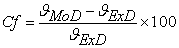(10)

Critical analysis of Figure 10, Figure 11 and Table 6 indicates that the evaluated correction factors are negative of the deviation as shown in equations (9) and (10).

The correction factor took care of the negligence of operational contributions of the surface properties of the clay and the physico-chemical interactions between the clay and the binder which actually played vital role during the water absorption process. The model predicted results deviated from those of the experiment because these contributions were not considered during the model formulation. Introduction of the corresponding values of Cf from equation (10) into the model gives exactly the corresponding experimental values of water absorption.

#### Table 6. Correction factor to model-predicted Water absorption

Table 6 also shows that the least and highest correction factor (to the model-predicted water absorption) are – 1.84 and - 7.09 %. Since correction factor is the negative of deviation as shown in equations (9) and (10), Table 6, Figure 10 and Figure 11 indicate that these highlighted correction factors corresponds to water absorptions: 16.9876 and 18.515%, Post-fired volume shrinkage: 25.63 and 24.82%, as well as apparent porosity: 21.9 and 22.48% respectively.

It is very pertinent to state that the deviation of model predicted results from that of the experiment is just the magnitude of the value. The associated sign preceding the value signifies that the deviation is a deficit (negative sign) or surplus (positive sign).

### 5. Conclusion

Following analysis of water absorption by the produced bricks while serving under submerged condition, it was concluded that water absorption decreases with decreasing apparent porosity and increasing post-fired volume shrinkage. The validity of the derived model (for water absorption analysis) was rooted in the core expression ξ – 107.6801 = - 1.0525 ₰ – 2.6392 ϑ where both side of the expression correspondingly approximately equal. The standard error incurred in predicting water absorption for each value of the post-fired volume shrinkage & apparent porosity considered, as obtained from experiment, derived model and regression model were 0.0704, 0.0693 and 4.38 x 10-5 & 0.0028, 0.2201 and 2.58 x 10-5 % respectively. Furthermore the correlation between water absorption and post-fired volume shrinkage & apparent porosity as obtained from experiment, derived model and regression model were all > 0.95. The maximum deviation of the model-predicted water absorption (from experimental results) was less than 8%. This translated into over 92% operational confidence for the derived model as well as over 0.92 synergic effective coefficients for the dependence of water absorption level of the submerged bricks on post-fired volume shrinkage & apparent porosity.

### References

  Barsoum, M., (1997) Fundamentals of Ceramics. McGraw Hill Incorporated, Singapore pp. 410.In article  Anwar, M. Y., Messer, P. F., Davies, H. A., Ellis, B. (1995). Ceramic Technology International 1996. Sterling Publications Ltd., London, pp. 95-96, 98.In article  Odriozola, A., Gutierrez, M., Haupt, U., Centeno, A. (1996). “Injection Moulding of Porcelain Pieces” Bol. Soc. Esp. Ceram. Vidrio 35 (2): 103-107.In article  Haupt, U. (1998). “Injection Moulding of Cups with Handles. International Ceramics. 2: 48-51.In article  Haupt, U. (2003). “Injection Moulding Technology in Tableware Production.” Ceramics World Review. 13 (54): 94, 96-97.In article  Rado, P. (1969).An Introduction to the Technology of Pottery. Pergamon Press.In article  Fortuna, D. (2000). ‘Sanitaryware Technology’ Gruppo Editoriale Faenza Editrice S.p.A.In article  Viewey, F. and Larrly, P. (1978). Ceramic Processing Before Firing, John-Wiley and Sons, New York, pp. 3-8.In article  Reed, J., (1988) Principles of Ceramic Processing,Wiley Interscience Publication, Canada pp. 470-478.In article  Keey, R.B. (1978). Introduction to Industrial Drying Operations, Pergamon Press, Elmsford, New York. pp. 132-157.In article  Nwoye, C. I. (2008). Mathematical Model for Computational Analysis of Volume Shrinkage Resulting from Initial Air- Drying of Wet Clay Products. Int. Res. J. Eng. Sc. & Tech. 5 (1): 82-85.In article  Nwoye, C. I., Iheanacho, I. O., Onyemaobi, O. O. (2008). Model for the Evaluation of Overall Volume Shrinkage in Molded Clay Products from Initial Air-Drying Stage to Completion of Firing. Int. J. Nat. Appl. Sc. 4 (2): 234-238.In article  Nwoye, C. I., Obidiegwu, E. O., and Mbah, C. N. (2014). Production of Bricks for Building Construction and Predictability of Its Post Fired Volume Shrinkage Based on Apparent Porosity and Water Absorption Capacity. Research and Reviews: Journal of Materials Science. 2 (3): 17-26.In article  BS EN ISO 10545-3: 1997.In article  Nwoye, C. I. (2008). C-NIKBRAN ‘‘Data Analytical Memory’’- Software.In article PubMed